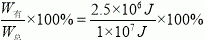# 质量为2.5t的小型载重汽车，额定功率为100kW，车上装有5t的砂石

12kg的汽油完全燃烧放出多少热量？（汽油的热值取4.5×107J/kg
2）汽车在平直公路上匀速行驶的功率为多少？
3）汽车从坡底向坡顶运送砂石的机械效率是多少？

1条回答
• 解析：

1）汽油燃烧放出的热量为：

Q=mq=2kg×4.5×107J/kg=9×107J

2）汽车的总重

G=mg=2.5+5×103kg×10N/kg=7.5×104N

阻力是：

f=0.2G=0.2×7.5×104N=1.5×104N

由于汽车匀速运动，则受到的牵引力为

F=f=1.5×104N

汽车在平直公路上匀速行驶的功率

P=Fv=1.5×104N×15m/s=2.25×105W

3）汽车在斜坡上做的有用功为：

W有用=Gh=mgh=5×103kg×10N/kg×50m=2.5×106J

总功

W=Pt=100000W×100s=1.0×107J

则机械效率为：

η==25%

故：

12kg的汽油完全燃烧放出9×107J热量；

2）汽车在平直公路上匀速行驶的功率为2.25×105W

3）汽车从坡底向坡顶运送砂石的机械效率是25%

讨论(1)看不清?